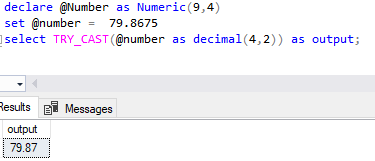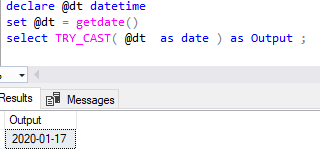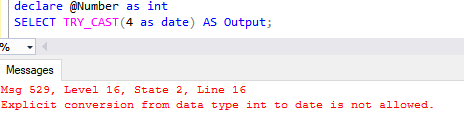Home » SQL SERVER TRY_CAST()

# SQL SERVER TRY_CAST()### SQL Server TRY_CAST() Function is a conversion functions that is used to cast a value to a specified data type.

It returns null if the conversion fails else returns the specified value in specified data type.

#### Syntax

`TRY_CAST ( expression AS datatype [ ( length ) ] )`

expression
The value to be cast.

datatype
The data type into which to cast expression.

length
Optional integer that specifies the length of the target data type.

Lets look at an example of TRY_CAST() in SQL.

#### Using SQL TRY_CAST() to Convert Numeric to Decimal

The following example uses the TRY_CAST() function to convert a numeric to a decimal.

```declare @Number as numeric(9,4)
set @number = 79.8675
select TRY_CAST(@number as decimal(4,2)) as output;```The following example uses the TRY_CAST() function to convert a date time to a date.

```declare @dt datetime

set @dt = getdate()

select TRY_CAST( @dt  as date ) as Output ;```The following example uses the TRY_CAST() function to convert a string to an integer.

```declare @txt as varchar(9)
set @txt ='5000'
select try_cast(@txt as int) as output```#### TRY_CAST() returns null when the cast fails

As you can see , Try_Cast returns null when we try to convert string text to Integer.

```declare @txt as varchar(9)
set @txt ='microsoft'
select try_cast(@txt as int) as output```If you try the same with CAST function, it will raise an error.

Lets do the same with CAST function and see what it returns.

```declare @txt as varchar(9)
set @txt ='microsoft'
select cast(@txt as int) as output```As you can see above , CAST function does not handle an error and returns conversion failed message.

It means when conversion fails , TRY_CAST() function returns null while CAST() function returns an error.

#### TRY_CAST() returns an error when the cast fails

TRY_CAST() function returns an error when the cast is explicitly not permitted.

Lets look into following statement, it returns an error when TRY_CAST is used to cast an integer value to date.

```declare @Number as int
select TRY_CAST(4 as date) as Output;```1,275 total views,  3 views today

This site uses Akismet to reduce spam. Learn how your comment data is processed.